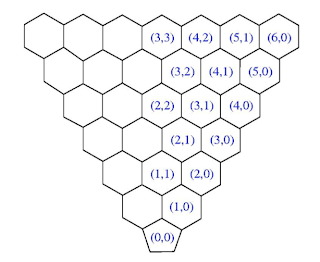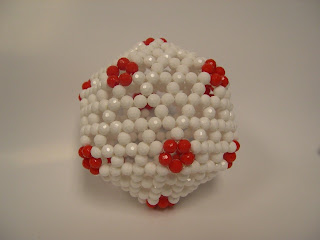## Friday, November 26, 2010

### Fullerenes belonging to icosahedral group

It is straightforward to make bead models for higher fullerenes with icosahedral symmetry. The simplest way is to use the Goldberg vector (see the following figure) to specify the relative position between two pentagons. Goldberg vector is very similar to the chiral vector used for defining carbon nanotubes.

Suppose we have the first pentagon located at orgin, (0,0), then we can ask where the next pentagon can we put? The answer is that any coordinate specified by (i,j) as shown in the following figure gives a unique fullerene with icosahedral symmetry. For instance, if the next pentagon is located at (i,j)=(1,1), we have a C60. It is not hard to show that the number of carbon atom for the fullerene specified by the Goldberg vector, (i,j), is N=20(i2 + ij + j2).(Figure 8 in "Jin, B.-Y.*; Chuang, C.; Tsoo, C.-C. “The Wonderful World of Beaded Molecules. 串珠分子模型的美妙世界” CHEMISTRY (The Chinese Chemical Society, Taipei) 2008, 66, 73-92, in chinese.")

A bead model of C60, Goldberg vector (1,1).Icosahedral fullerene specified by the Goldberg vector (2,1) has 140 carbon atoms. This is the smallest chiral fullerene with icosahedral symmetry.The following beaded fullerene is specified by (4,0) has 320 carbon atoms.